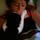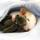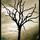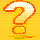## General Question# Brain Teaser - Can you complete this proof that everyone (yes, including you) ought to know?

Asked by LostInParadise (28552) April 19th, 2009

In every subject there are certain things that should be known by the general public. There are certain paintings, novels and poems that an educated person should be familiar with, though this knowledge may not make you wealthier or improve your love life. I say the same is true of mathematical proofs.

This brain teaser is based on a very short and very sweet proof due to Euclid that shows there are infinitely many prime numbers. I will start you off. See if you can finish and thereby receive an aha! moment.

The proof is usually presented as indirect, but I think that is an unnecessary complication. The proof is constructive, meaning that you can use it, at least in theory, to find a prime number larger than any given number.

Given a number n, consider all the primes p1,...,pk that are less than or equal to n. Actually finding these primes is a bit of work, but it can always be done in a finite amount of time. Now multiply all these primes together and add 1. That is, create the number m=p1*p2*...*pk + 1. Why does this number m show that there is a prime greater than n?

Please don’t spoil if for others. You can send me a PM with the answer or, if you prefer, explain why you do not see any way that knowing this proof will make you a better person. I will give the answer in two days.

Observing members: 0Composing members: 0or, if you prefer, explain why you do not see any way that knowing this proof will make you a better person

I engage in a utilitarian viewpoint of “better” or “worse.” This has no application to my life and my life will not be measurably improved by knowing this proof. By virtue of this information being obscure, impractical, and irrelevant, I contest that I will in no way be a “better” person for knowing it.

In fact, I would say my life has been measurably worsened by being told that I “ought to know” how to solve this.

That said, I think I do have a solution. Why don’t you explain to me why you think I should share it with you?

nikipedia (27726)“Great Answer” (3) Flag as…Mother Teresa could have been a better person for knowing this, but she obstinately chose to disregard it. Consequently, she went to Hell.

ratboy (15157)“Great Answer” (2) Flag as…Where did you get your list of things that “educated people ought to know”? Speak for yourself.

BBSDTfamily (6834)“Great Answer” (0) Flag as…The proof is constructive, meaning that you can use it, at least in theory, to find a prime number larger than any given number.

The proof is not constructive; it demonstrates that there is a prime greater than n, but yields no algorithm for producing such a prime. Filip Saidak found a simple constructive proof in 2005.

ratboy (15157)“Great Answer” (1) Flag as…@BBSDTfamily , It might be interesting to open a thread to discuss what it means to be an educated person. There is too much knowledge for someone to be a Renaissance man (or woman), but I think there is some core of knowledge an educated person should have in a variety of fields. I would be curious to see your view.

@ratboy , Thanks for showing the new proof. I still maintain that Euclid’s proof can be made constructive. Just factor m. There is no efficient algorithm for doing this, but even the most brute force method will produce a new prime in a finite period of time. That shows that for any n, there is a prime greater than n. Repetition of the procedure will produce an infinite sequence of primes.

LostInParadise (28552)“Great Answer” (0) Flag as…What I’m going to say here is not much relevant to the question, per se, but I find it interesting. I first saw a part of it a while back in an article and I was able to generalize it.

If you look at p1…pk + 1, it has a prime factor that is none of p1,..., pk. Now the question is, can we remove one of these pi’s and still claim that there is a new prime factor?

For example if we remove pi, can we say the product of p1, through pk except for pi + 1, has a prime factor that is not pi? The answer is yes, except in a few “small” counter examples.

The argument needs a bit of work but here is a gist of how it starts. If somebody is interested I can elaborate more:

Let a = (the product of p1,...,pk except for pi) + 1. If there is no new prime factor then all of its prime factors should be pi, which means a = pi^m.

Now we can show that m > 1.

And it can be shown that a can never be m-th power of any integer, in particular pi.

P.S. You can use this fact to show the (2n)-th prime number is less than or equal to the product of the first n prime numbers plus 1.

BonusQuestion (1483)“Great Answer” (0) Flag as…@BonusQuestion, I would be curious to see why a can not be the mth power of any integer.

The answer to the brain teaser is as follows. The number m, when divided by any of the pi, will have a remainder of 1 and therefore is not divisible by any of the pi. But we know that any number can be expressed as the product of primes, so m must be divisible by some prime. Since we know that it is not divisible by any of the pi and the pi are all the primes less than or equal to n, then m must be divisible by some prime greater than n.

LostInParadise (28552)“Great Answer” (0) Flag as…If you start at the beginning, the numbers m generated start out as all primes.

2 + 1=3
2 * 3 + 1 = 7
2 * 3 * 5 + 1 = 31
2 * 3 * 5 * 7 + 1 = 211
2 * 3 *5 *7 *11 + 1 = 2311

It is only when you reach 13 that you first get a non-prime, all of whose factors must of course be greater than 13.
2 × 3 × 5 × 7 × 11 × 13 + 1 = 30,031 = 59 × 509

LostInParadise (28552)“Great Answer” (0) Flag as…It appears that I cannot show a is not m-th power of any integer. However I can still show it is not m-th power of pi. (Which is what I need for my previous claim). In just the last step I need to assume x=pi. So I don’t assume x=pi until the very last line of the argument.

The proof is a bit long. So I am trying to break that into a few steps.

First lets prove this lemma.

~~~~~~~~~~~~~~~~~~~~~~~~~~~~~~~~~~~

Lemma. Let p and q be two prime numbers, x and m be two positive integers such that x^m – 1 is divisible by q and m is divisible by p. Then one of the following two cases occures.

1) x^(m/p) = 1 (mod q)

2) p divides q-1

Proof of this lemma goes like this: m is divisible by p. So if we let b = x^(m/p), this is an integer and b^p – 1 is divisible by q.
On the other hand, by Fermat’s Little Theorem, we know that b^(q-1) – 1 is divisible by q.

Now if r is g.c.d. of p and q-1, since we can write r as a linear combination of p and q-1 and then use the following two facts:

b^p = 1 (mod q)

&

b^(q-1) = 1 (mod q)

And deduce that: b^r = 1 (mod q).

Now, since r divides p it is either 1 or p.

1) r=1, by the above equation we have b = 1 (mod q) which is what we wanted to prove.

2) r=p. This means p divides q-1 which is the other case of the Lemma.

~~~~~~~~~~~~~~~~~~~~~~~~~~~~~~~~~~~~~

Now, back to the original statement. Let a = (the product of p1,..., pk excluding pi) + 1 = x^m. Therefore we have x^m = 1 (mod pj) for j <= k and j <> i.

Since m > 1, it has a prime factor p. Now I am trying to show this is impossible by checking different assumptions on p.

Case 1) p > pk. We have x^m = 1 (mod pj). By lemma either p divides pj – 1 or x^(m/p) = 1 (mod pj). But since pj – 1 is less than p, the first case is impossible. Therefore x^(m/p) = 1 (mod pj).

Hence x^(m/p) – 1 is divisible by (the product of p1,..., pk excluding pi) which was a – 1 = x^m -1. This is a contradiction, because x^(m/p)-1 is less than x^m – 1.

Case 2) p = pj for some j < k and j<>i. Since p is one of pj’s, we have x^m = 1 (mod p) and p divides m. Therefore, by Lemma, either p divides p-1 or x^(m/p) = 1 (mod p). The first one is impossible so x^(m/p) = 1 (mod p).

But if you take y = x^(m/p) then we have x^m – 1 = y^p – 1 = (y-1) (y^(p-1)+....+y+1).

Since y = 1 (mod p) for the second expression we have: (y^(p-1)+....+y+1) = 1+1+...+1 = p = 0 (mod p). Hence (y^(p-1)+....+y+1) is divisible by p. Therefore y^p-1 is divisible by p^2. So x^m – 1 is divisible by p^2. But x^m-1 is square free which is a contradiction.

Case 3) p=pi (This is the same i that we excluded from the product.). The above arguments showed that all of prime factors of m are pi. So we can assume m = pi^l.

Now I know x^m = 1 (mod pj) for any j <= k and j<>i. So by Lemma either pi divides pj-1 or x^(m/pi) = 1 (mod pj).

If we only look at j < i, the first case cannot occure. So we have x^(m/pi) = 1 (mod pj) for j < i. Repeat this argument and we can reduce the exponent of x, which is m/pi to m/pi^2. Repeating this argument we can finally get rid of m and get x = 1 (mod pj). Which means x – 1 is divisible by p1…p(i-1)

It appears that only for this part of my argument I need to assume x = pi. If x=pi this cannot happen as one can pretty easily show pi < p1….p(i-1)+1.

~~~~~~~~~~~~~~~~~~~~~~~~~~~~~~~~~~~~

This is a variation of the proof presented in the following article. (I thought I could drop the condition x=pi by modifying the proof, but apparently I can’t!)

Cosgrave, John B. A remark on Euclid’s proof of the infinitude of primes. Amer. Math. Monthly 96 (1989), no. 4, 339—341.

P.S. This argument shows that p1…pk + 1 (which is sometimes called Euclid’s number) cannot be the m-th power of any number.

BonusQuestion (1483)“Great Answer” (1) Flag as…I was looking at wikipedia article about Euclid Numbers. It says ”A Euclid number can not be a perfect square.” Perhaps one of us should add that it cannot be the m-th power for any m>1. :)

BonusQuestion (1483)“Great Answer” (0) Flag as…or